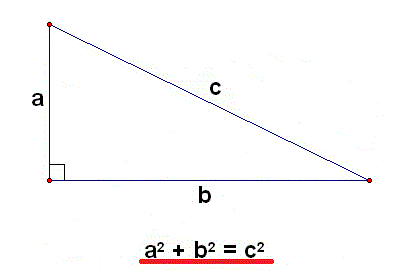# Detailed Right Triangle (◺) Pythagorean Theorem Calculation#A Step By Step Pythagorean Theorem Calculator

An online detailed geometry pythagoreas calculator along with step by step calculation.

Step by Step calculation## Pythagorean Theorem Formula

a2 + b2 = c2

For example: To Find A, Enter b=7, c=9, Answer is A= 5.66)The Pythagorean Theorem is the statement that the sum of (the areas of) the two small squares equals (the area of) the big one.

In math algebraic terms, a2 + b2 = c2 where c is the hypotenuse while a and b are the legs of the triangle.

###Related Calculator

Distance Between Two Points

Distance Between Two MidPoints

Geometric Progression Calculator

Arithmeic Progression Calculator

###More Calculators

Short Distance Between Two Lines

Geometric Mean Calculator

More >>##Age Calculators ►

Horse Age Calculator , Cow Age Calculator , Cat Age Calculator , Dog Age Calculator, Human Age Calculator 100 Days Old Calculator Date Difference Calculator